# Angle + prism - math problems

#### Number of problems found: 23What is the volume of a quadrilateral oblique prism with base edges of length a = 1m, b = 1.1m, c = 1.2m, d = 0.7m, if a side edge of length h = 3.9m has a deviation from the base of 20° 35' and the edges a, b form an angle of 50.5°.
• Water channelThe cross section of the water channel is a trapezoid. The width of the bottom is 19.7 m, the water surface width is 28.5 m, the side walls have a slope of 67°30' and 61°15'. Calculate how much water flows through the channel in 5 minutes if the water flo
• Paper box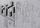Calculate the consumption of paper on the box-shaped quadrangular prism with rhombic footstall, base edge a=6 cm and the adjacent base edges forms an angle alpha = 60 °. Box height is 10 cm. How many m2 of the paper consumed 100 such boxes?The height of a regular quadrilateral prism is v = 10 cm, the deviation of the body diagonal from the base is 60°. Determine the length of the base edges, the surface, and the volume of the prism.Calculate the volume (V) and the surface (S) of a regular quadrilateral prism whose height is 28.6 cm and the deviation of the body diagonal from the base plane is 50°.
• Octagonal tank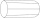The tank has the shape of a regular octagonal prism without an upper base. The base edge has a = 3m, the side edge b = 6m. How much metal sheet is needed to build the tank? Do not think about losses or sheet thickness.
• Four sided prismCalculate the volume and surface area of a regular quadrangular prism whose height is 28.6cm and the body diagonal forms a 50-degree angle with the base plane.
• Pentagonal prismThe regular pentagonal prism is 10 cm high. The radius of the circle of the described base is 8 cm. Calculate the volume and surface area of the prism.
• Office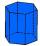Office building was built in the shape of a regular hexagon inscribed in a circle with a radius of 12 m. The height of the walls is 7m. How much CZK cost plastering the walls of the building, if per 1 m square cost CZK 400?
• Prism diagonalThe body diagonal of a regular square prism has an angle of 60 degrees with the base, the edge length is 10 cm. What is the volume of the prism?
• DiagonalDetermine the dimensions of the cuboid, if diagonal long 53 dm has an angle with one edge 42° and with another edge 64°.
• Angle of diagonalThe angle between the body diagonal of a regular quadrilateral and its base is 60°. The edge of the base has a length of 10cm. Calculate the body volume.
• Hexagonal prismThe base of the prism is a regular hexagon consisting of six triangles with side a = 12 cm and height va = 10.4 cm. The prism height is 5 cm. Find the volume and surface of the prism.
• GlassHow many glass are needed to produce glass with base regular 5-gon if one base triangle in the base is 4.2 square cm and the height is 10 cm?
• Triangular prismThe plane passing through the edge AB and the center of segment CC' of regular triangular prism ABCA'B'C', has an angle with base 22 degrees, |AB| = 6 cm. Calculate the volume of the prism.
• Decagon prism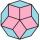A regular decagon of side a = 2 cm is the base of the perpendicular prism, the side walls are squares. Find the prism volume in cm3, round to two decimal places.
• Diagonals of a prismThe base of the square prism is a rectangle with dimensions of 3 dm and 4 dm. The height of the prism is 1 m. Find out the angle between the body diagonal with the diagonal of the base.
• Hexagonal prism 2The regular hexagonal prism has a surface of 140 cm2 and height of 5 cm. Calculate its volume.
• Triangular prismCalculate the surface of a regular triangular prism, the edges of the base are 6 cm long and the height of the prism is 15 cm.
• The rod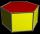The rod has the shape of a regular hexagonal prism with a volume of 32.4 cubic decimetres. What is the content of the base if it is 350 centimeters long? Round to ones.

Do you have an exciting math question or word problem that you can't solve? Ask a question or post a math problem, and we can try to solve it.

We will send a solution to your e-mail address. Solved examples are also published here. Please enter the e-mail correctly and check whether you don't have a full mailbox.

Angle Problems. Prism Problems.